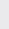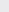Course Content

0/4

0/3

0/1

0/2

0/1

0/4

0/3

0/5

0/3

0/5

0/5

0/1

0/6

0/2

0/4

0/5

12.3 Parallel and Perpendicular Between Two Lines 两条直线的平行与垂直

(1) The lines+=1and 4x + 3y – 4 = 0 are parallel, find the value of t and the distance of the lines.

2, 1
(2)Points A(-1, 4), B(2, 7), C and D(1, 0) are the vertices of a parallelogram. Point E lies on BC such that BE =BC. The lines that parallel to y-axis is drawn from A, meet x-axis at N and from E, meet line CD at F.

(a) Calculate coordinate of C and E.

(b) Find the equation of line DC and determine coordinate of F.

(c) Explain why AEFN is a parallelogram and calculate its area.

(a) (4, 3), (2, 5)

(b) y = x – 1, (2, 1)

(c) 14unit(3)
A, B and C are three points on line 2y + x = 10 that meets y-axis at A with AB : BC = 3 : 2 and point C is (8, p). Find
A, B 和 C 是直线 2y + x = 10 上的三个点并交 y-轴于 A 使得 AB : BC = 3 : 2 且点 C 是 (8, p). 求

(a) coordinate of point A,
A 点坐标

(b) value p,
p 的值，

(c) coordinate of point B,
B 点坐标，

(d) equation of the line that passes through point C and perpendicular to AC.